Maths Index

Mathematics Formulae

Note: For Series refer to Series

Mensuration

Area of a Triangle, (sides a,b,c).. Area = ( b . c sin A )/ 2

Area of a Triangle = Sqrt (s .(s - a). (s - b). (s - c))........( s = (a + b + c)/ 2.)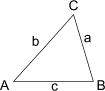Area of a Circle (r = radius).. Area = π . r 2

Area and Volume of a Cylinder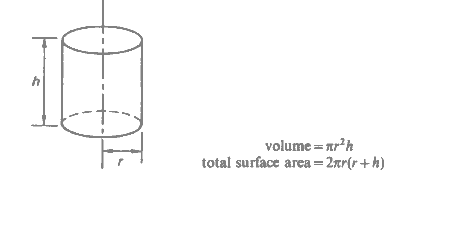Area and Volume of a Cone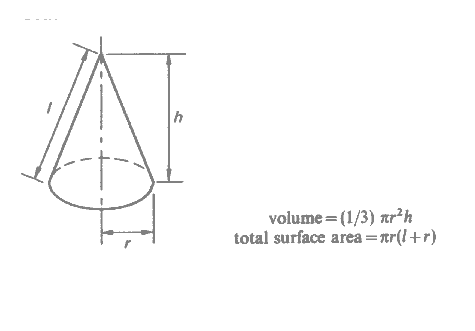Area and Volume of a Frustrum of a Cone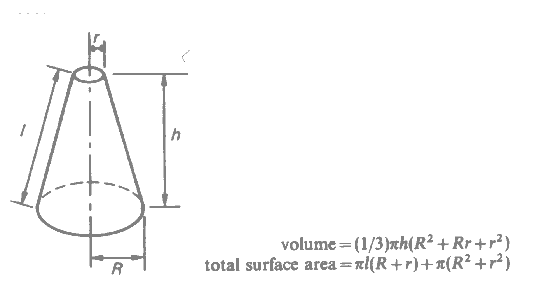Area and Volume of a Sphere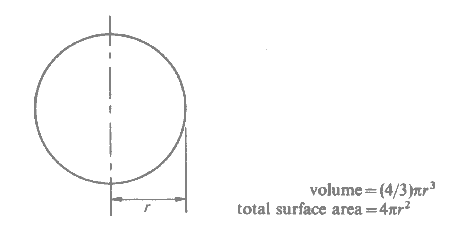Area and Volume of a Pyramid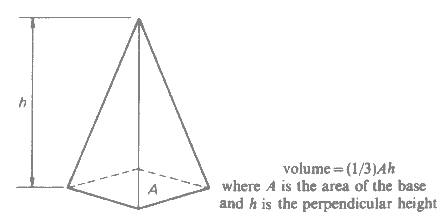Trigonometry...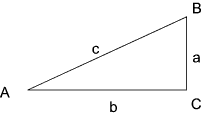Definitions... Sin A = Opposite / Hypotenuse = a / c Cosine A = Adjacent / Hypotenuse = b / c Tangent A = Opposite / Adjacent = a / b Cosecant A = 1 / sin = c / a Secant A = 1/cosine = c / b Cotangent A = 1/tangent. = b / a Trigonometric Relations... Sin ( - A) = - Sin (A) Cos ( - A) = cos (A) Sin (A) 2 + Cos A 2 = 1 Cos (A) 2 =(1 + Cos (2A) ) /2 Trigonometric Relations...(continued) Sin (A) 2 =(1 - Cos (2A) ) /2 Sin (A) Cos(A) = Sin (2A) /2 Sin (A + B) = Sin (A) Cos(B) + Cos(A) Sin(B) Sin (A - B) = Sin (A) Cos(B) - Cos(A) Sin(B) Cos (A + B) = Cos (A) Cos(B) - Sin(A) Sin(B) Cos (A - B) = Cos (A) Cos(B) + Sin(A) Sin(B) Sin(A).Sin(B) = [-Cos(A+B) + Cos (A-B)]/2 Cos(A).Cos(B) = [Cos(A+B) + Cos (A-B)]/2 1 + tan(A) 2 = sec(A) 2 1 + cot(A) 2 = cosec(A) 2

Hyperbolic Functions
 sinh x = (e x - e - x) / 2 cosh x = (e x + e - x) / 2 tanh x = sinh x / cosh x = (e x - e - x) / (e x + e - x) sech x = 1 / sinh x = 2 / (e x - e - x) cosech x = 1 / cosh x = 2 / (e x + e - x) coth x = cosh x / sinh x = (e x + e - x) / (e x - e - x) ejx = cos x + j sin x ex = cosh x + j sinh x sin x = (e jx - e - jx) /2.j cos x = (e jx + e - ix) /2 sin jx = j.sinh x cos jx = cohs x

A quadratic equation is generally of the form...

ax2 +b x + c = 0

The general solution of this equation is

x = ( - b ± Ö (b2 - 4 a c ) /2a

Expansions

The expansions below are developed using Taylors theorem

Trigonometric Expansions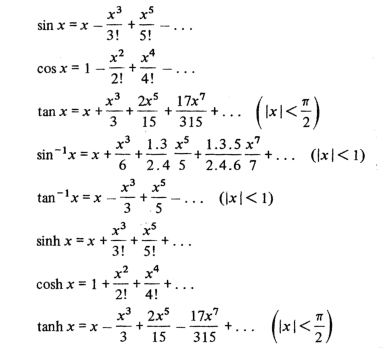Exponential /Logarithmic Expansions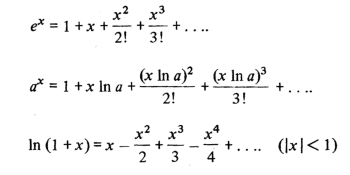Derivatives..
 f (x) f '(x)= df(x) / dx sin x cos x cos x - sin x tan x sec2 x cotan x - cosec2 x sec x sec x. tan x cosec x ... - cosec x. cot x. sinh x ... cosh x. cosh x ... sinh x. tanh x ... sech2 x. cosech x ... - coth x cosech x sech x ... - tanh x sech x coth x ... - cosech 2 x u .v u . dv/dx + v . du/dx u / v (v . du / dx - u . dv / dx ) / v 2 a. x n a. n . x n - 1 e a x a . e a x a x a x. ln a x x x x /(1 + ln x) ln x 1 / x log a x 1 / x . log a e sin - 1( x /a) 1 / Sqrt(a 2 - x 2 ) cos - 1( x /a) - 1 / Sqrt(a 2 - x 2 ) tan - 1( x /a) a / (a 2 + x 2 )

Indefinite Integrals.....Integration by Parts

y = f(x) ...y is a function of x

F(x) is the integral of f(x)

The differentiation of F(x) results in f(x)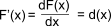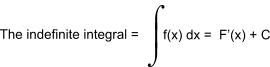On differential the unknown constant C disapears.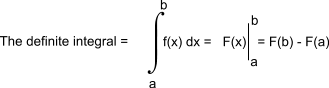Table of Indefinite Integrals

 f(x)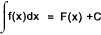The constant of integration C is ommitted from the table of indefinite integrals below xa x a+1 / (a + 1) 1 / (x 2 + a2) (1 / a) . tan - 1 (x / a) 1 / (x 2 - a2) (1 /2 a) . ln ( ( x - a ) /(x + a)) ( a + b x ) n   (n not - 1) (a + b x)n + 1 / b (n + 1) ( a + b x ) - 1 1 / b .ln ( a + b x ) x / (a x +b) (a x + b - b ln(ax +b) ) / a2 1 / x ln x 1 / Sqrt (x 2 - a 2) cosh - 1 (x / a) 1 / Sqrt (x 2 + a 2) sinh - 1 (x / a) ex ex 1 / ( a2 - x2) (1 / a). tanh - 1 ( x / a ) = 1 /( 2. a) . log(a + x/a - x ) ax ax / ln a x ax (a x / ln a ) - (a x /( ln a ) 2 ) x ea x e a x (a x - 1) / a2 1 /(a + b e c x ) (x / a) - ln (a + b ec x ) / a c ln x x (ln x - 1 ) ( ln x )2 x [ (ln x )2 - 2 ln x +2 ] 1 / x ln x ln ( ln x ) sin x - cos x cos x sin x tan x - ln cos x cotan x ln sin x sec x ln ( sec x + tan x ) = ln (tan (x/ 2 + π/ 4) ) cosec x log | tan x/ 2 | 1 / Sqrt( x 2 + a 2) sinh - 1( x / a ) = log ( (x/a) +Sqrt(x2 /a2 +1)) 1 / Sqrt( x2 - a 2) cosh - 1( x / a ) 1 / Sqrt( a2 - x 2) sin - 1( x / a ) sinh x cosh x cosh x sinh x tanh x ln cosh x cosech x ln tanh (x / 2 ) sech x tan - 1 ( sinh x ) coth x ln sinh x sinh 2 x ( - x + ( sinh (2 x)) /2 ) / 2 cosh 2 x ( x + ( sinh (2 x) ) /2 ) / 2 sech 2 x tanh x cosech 2 x - coth x tanh 2 x x - tanh x

Moments Of Inertia of Plane Sections

I = moment of Inertia about the identified axis.
J = Polar moment of inertia about the centroid of section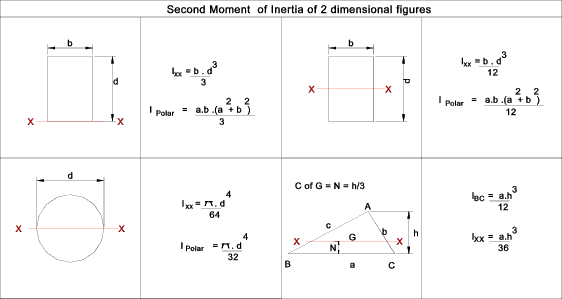For More detailed information refer..

Parallel axis Theory..

If the second moment of an area (A) about an axis x - x = I xx. Then the second moment of Area about a parallel axis y - y which is distance x from x - x =

I yy = I xx + A . x 2

Complex Numbers..

In mathematics it is necessary to provide a method of identifying the root of a negative number i.e p = ( - 4).   p is clearly not real number it is an imaginary number.

Again an equation x2 - 2x +5 = 0 results in (x - 1)2 = - 4 so that (x - 1) = ± ( - 4).   The roots are therefore x = 1 - ( - 4), and 1 + ( - 4),   These roots which are a combination of a real number and an imaginary number are called complex numbers.

The symbol i (j in electical work) is used to represent - 1.   Therefore ( - 4) = 2i.  The number i, or 1i , or xi are called purely imaginary numbers.

The complex number solution of the above equation = and 1 + 2i and 1 - 2i,

Powers of complex are identified below

 i 1 = +i i - 1 = - i i 2 = - 1 i - 2 = - 1 i 3 = - i i - 3 = +i i 4 = +1 i - 4 = - 1 ... ... i... ... i 5 = +i i - 5 = - i

etc .

For two complex numbers (a 1 + ib 1) & (a 2 +ib 2)to be equal it can be easily proved that a 1 must equal a 2, and b 1 must equal b 2

Complex numbers are conveniently represented using an argand diagrams as shown below.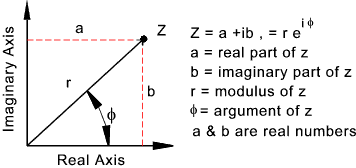Complex numbers can be manipulated using the Cartesian system as follows;

z = a + i b

z 1 + z 2 = (a 1 + a 2) + i (b 1 + b 2)

z 1 - z 2 = (a 1 + a 2) - i (b 1 + b 2)

z 1 . z 2 = (a 1 . a 2 - b 1 .b 2) + i (a 1 . b 2 + a 2 .b a)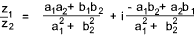a2 + a2 = r2 = (a +ib) (a - ib)

Complex numbers can be manipulated using the polar co - ordinate system as follows;

z = a + i b = r (cos φ + i. sin φ )

r = (a2 + b2 ),

φ = arctan (b/a) = tan - 1 (b/a)

sin φ = b/r,    cos φ = a/r,   tan φ = b/a

z 1. z 2 = r 1.r 2 [cos (φ 1 + φ 2 ) + i (sin (φ 1 + φ 2 ) ]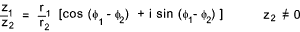z n = r n [cos (n φ) + i sin (n φ) ]       z > 0 , Integer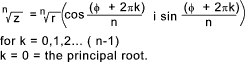e i φ = cos ( φ) + i sin ( φ )    Eulers formula

i φ =ln [ (cos ( φ) + i sin ( φ ) ]

e - i φ = cos ( φ) - i sin ( φ ) = 1/ [ cos ( φ) + i sin ( φ ) ]

 Useful Related Links Platonic Realms ...Very Extensive Mathematics Information /formulae. The Maths Pages.. Easy to follow mathematics notes. Scienceworld.wolfram .... Welcome to the best resource of Science and math on the Internet - Probably true Karls Calculus Tutor .... This is an excellent site for learning Calculus
Maths Index